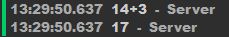# How should i do this?

basically, i have a table of very simple math problems: `local EasyProblems = {17+10, 20+13, 40-21, 20-7, 14+12, 18+2, 21-3, 14-8, 15+6, 9+10, 7+7, 14+3, 14+8, 19-3, 14-2, 10-10, 0-7, 7+9, 17-8, 17-13}`
and with this: `local RandomEasyProblem = EasyProblems[math.random(1, 19)]` it can choose the answer to a random problem. however, i want the problem to appear in a TextLabel and then the player would have to type the answer to the chosen problem in a TextBox, or the answer is a `local` variable.

CODE:

``````local Main = script.Parent
local Frame = Main:WaitForChild("Frame")
local Text = Frame:WaitForChild("TextLabel")
local EasyProblems = {17+10, 20+13, 40-21, 20-7, 14+12, 18+2, 21-3, 14-8, 15+6, 9+10, 7+7, 14+3, 14+8, 19-3, 14-2, 10-10, 0-7, 7+9, 17-8, 17-13}
local RandomEasyProblem = EasyProblems[math.random(1, 19)]
print(RandomEasyProblem)
Text.Text = RandomEasyProblem
``````
2 Likes
``````local Main = script.Parent
local Frame = Main:WaitForChild("Frame")
local Text = Frame:WaitForChild("TextLabel")

local EasyProblems = {"17+10", "20+13", "40-21", "20-7", "14+12", "18+2", "21-3", "14-8", "15+6", "9+10", "7+7", "14+3", "14+8", "19-3", "14-2", "10-10", "0-7", "7+9", "17-8", "17-13"}
local RandomEasyProblem = EasyProblems[math.random(1, #EasyProblems)]

print(RandomEasyProblem)
Text.Text = RandomEasyProblem
``````

I made them into strings, should work now

3 Likes

ive already tried that but i think you forgot this:

or when the problem shows up the answer to it would be printed?

1 Like

Just as some advice, if you’re checking the answer too, convert it to a number at it will give you the answer.

You can do this with `string.split()` and `string.match()`, I simplified it in a function.

``````local Seed = Random.new()

local Splited
if Problem:match("+") then
Splited = string.split(Problem, "+")
elseif Problem:match("-") then
Splited = string.split(Problem, "-")
end
end

local Main = script.Parent
local Frame = Main:WaitForChild("Frame")
local Text = Frame:WaitForChild("TextLabel")

local EasyProblems = {"17+10", "20+13", "40-21", "20-7", "14+12", "18+2", "21-3", "14-8", "15+6", "9+10", "7+7", "14+3", "14+8", "19-3", "14-2", "10-10", "0-7", "7+9", "17-8", "17-13"}
local RandomEasyProblem = EasyProblems[Seed:NextInteger(1, #EasyProblems)]

print(RandomEasyProblem)
Text.Text = RandomEasyProblem
``````2 Likes

Why bother doing that when if you convert it, lua will automatically give the answer without any of that necessary.

Please do some research before making comments, if `tonumber()` finds a character which is not a number it will automatically return nil.

``````local Problem = "2+3"

print(tonumber(Problem)) -- nil
``````

Ah right, I didn’t consider that, totally slipped my mind.

``````local Main = script.Parent
local Frame = Main:WaitForChild("Frame")
local Text = Frame:WaitForChild("TextLabel")

function RNG(Min, Max)
return Random.new():NextInteger(Min, Max)
end

function GetOperator()
local Operators = {
"+",
"-"
}

return Operators[RNG(1, #Operators)]
end

function Solve(Problem)
local First, Operator, Last = string.match(Problem, "(%d+)(.-)(%d+)")
local Operators = {
["+"] = function()
return First + Last
end,
["-"] = function()
return First - Last
end
}

return Operators[Operator]()
end

function CreateProblem()
local Problem = RNG(0, 100)..GetOperator().. RNG(0, 100)

repeat
Problem = RNG(0, 100)..GetOperator().. RNG(0, 100)
until Solve(Problem) % 1 == 0

return Problem, Solve(Problem)
end

Text.Text = Problem
``````

this code only changes the text label once, if you want to make the questions go on forever you need to have something to submit the answer with(like a button)

the code gives random problems and answers so you don’t have to

this won’t work for subtraction
`GetAnswer("10-5")` returns 15 not 5

this is because of the last line, you always add the numbers

so which one is the one that will actually work?

my code works, but if you read you will have to do more if you want multiple problems

I didn’t implement it because I don’t know how your explorer looks or how you want it to work

how come this doesnt work?

``````TextType.FocusLost:Connect(function(enterpressed)
if enterpressed and TextType.Text == Answer then
print("e")
end
end)
``````

“e” prints if the if statement changes to: `if enterpressed and TextType.Text ~= Answer then`

also how would i do different modes of difficulty as the functions are adding and subtraction, for example the function “CreateProblem” could be “CreateEasyProblem” and then there is another function like that but it is “CreateHardProblem” which is something else. to do that would there have to be more functions then different CreateProblem functions?

what would a hard problem look like?

what happens when you print TextType.Text?

probably something like: (𝑥^3)^2(𝑦^5)^2
basically multiplying and dividing monomials
and it prints what i typed

yea my bad, I know why it doesn’t work now
you are comparing a string and a number

``````TextType.FocusLost:Connect(function(enterpressed)
if enterpressed and tonumber(TextType.Text) == Answer then
print("e")
end
end)
``````

we can fix this by using `tonumber()`

1 Like

i thought so, knew there was a function to do that but didnt know what it was

well ill experiment around with stuff and if i have a question ill just dm you, thanks

1 Like